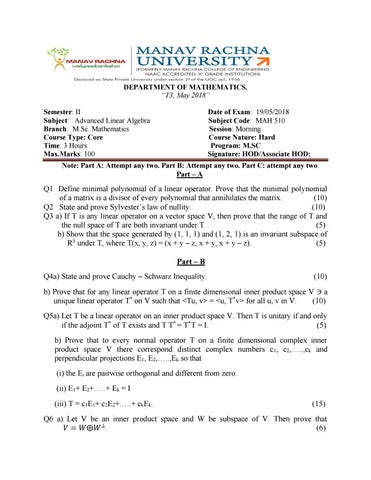DEPARTMENT OF MATHEMATICS. â&#x20AC;&#x153;T3, May 2018â&#x20AC;? Semester: II Subject: Advanced Linear Algebra Branch: M.Sc. Mathematics Course Type: Core Time: 3 Hours Max.Marks: 100

Date of Exam: 19/05/2018 Subject Code: MAH 510 Session: Morning Course Nature: Hard Program: M.SC Signature: HOD/Associate HOD:

Note: Part A: Attempt any two. Part B: Attempt any two. Part C: attempt any two. Part â&#x20AC;&#x201C; A

Q1 Define minimal polynomial of a linear operator. Prove that the minimal polynomial of a matrix is a divisor of every polynomial that annihilates the matrix. (10) Q2 State and prove Sylvesterâ&#x20AC;&#x2122;s law of nullity. (10) Q3 a) If T is any linear operator on a vector space V, then prove that the range of T and the null space of T are both invariant under T. (5) b) Show that the space generated by (1, 1, 1) and (1, 2, 1) is an invariant subspace of R3 under T, where T(x, y, z) = (x + y â&#x20AC;&#x201C; z, x + y, x + y â&#x20AC;&#x201C; z). (5) Part â&#x20AC;&#x201C; B Q4a) State and prove Cauchy â&#x20AC;&#x201C; Schwarz Inequality.

(10)

b) Prove that for any linear operator T on a finite dimensional inner product space V â&#x2C6;&#x2039; a unique linear operator T* on V such that <Tu, v> = <u, T*v> for all u, v in V. (10) Q5a) Let T be a linear operator on an inner product space V. Then T is unitary if and only if the adjoint T* of T exists and T T* = T*T = I. (5) b) Prove that to every normal operator T on a finite dimensional complex inner product space V there correspond distinct complex numbers c1, c2,â&#x20AC;Ś..,ck and perpendicular projections E1, E2,â&#x20AC;Ś..,Ek so that (i) the Ei are pairwise orthogonal and different from zero. (ii) E1+ E2+â&#x20AC;Ś..+ Ek = I (iii) T = c1E1+ c2E2+â&#x20AC;Ś..+ ckEk.

(15)

Q6 a) Let V be an inner product space and W be subspace of V. Then prove that đ?&#x2018;&#x2030; = đ?&#x2018;&#x160;â¨ đ?&#x2018;&#x160; â&#x160;Ľ. (6)

b) If in an inner product space ||u + v|| = ||u|| + ||v||, then prove that the vectors u and v are linearly dependent. (4) c) Prove that any orthonormal set of vectors in an inner product space is linearly dependent. (5) d) Apply the Gram Schmidt process to the vectors (1, 0, 2, 0) , (1, 2, 3, 1), to obtain an orthonormal basis for R4 with the standard inner product. (5) Part â&#x20AC;&#x201C; C Q7a) If U is an n â&#x20AC;&#x201C; dimensional vector space with basis {u1, u2,â&#x20AC;Śun}, if V is an m â&#x20AC;&#x201C; dimensional vector space with basis {v1, v2,â&#x20AC;Śvm}, and if {aij} is any set of nm scalars (i = 1, 2,â&#x20AC;Śn; j = 1,â&#x20AC;Śm) then there is one and only one bilinear form on đ?&#x2018;&#x2C6;â¨ đ?&#x2018;&#x2030; such that f(ui, vj) = aij for all i and j. (8) b) Let F be the bilinear form on R2 defined by f((x1,x2), (y1, y2)) = (x1+x2) (y1+y2). Find the matrix of f in the ordered basis B = {(1, -1), (1, 1)}. (3) c) Is the bilinear form 2x1y â&#x20AC;&#x201C; x1y2 + x2y2 â&#x20AC;&#x201C; x3y3 symmetric ? Validate your answer. (2) 1 d) Reduce the form đ?&#x2018;&#x2039; đ??´đ?&#x2018;&#x152; into canonical form where đ??´ = [ 3 â&#x2C6;&#x2019;1 the equations of transformations. â&#x20AC;˛

0 1 â&#x2C6;&#x2019;1

â&#x2C6;&#x2019;2 â&#x2C6;&#x2019;3]. Also find â&#x2C6;&#x2019;1 (7)

Q8 a) Prove that every bilinear form on the vector space V over a subfield F of the complex numbers can be uniquely expressed as the sum of a symmetric and skew symmetric bilinear forms. (8) b) Diagonalize the quadratic form x12 â&#x20AC;&#x201C; 4x22 + 6x32 + 2x1x2 â&#x20AC;&#x201C; 4 x1x3 + 2x42 â&#x20AC;&#x201C; 6 x3x4. Find the equations of linear transformations. Also find the rank, index and signature of the form.

(12)

Q9 a) Let f be a non â&#x20AC;&#x201C; degenerate bilinear form on a finite dimensional vector space V. Prove that the set G of all linear operators on V which preserve f is a group under the operation of composition. (8) b) Show that the quadratic form - 21 x12 + 30x1x2 â&#x20AC;&#x201C; 12 x1x3 â&#x20AC;&#x201C; 11x22 + 8x2x3 â&#x20AC;&#x201C; 2 x32 is negative semi â&#x20AC;&#x201C; definite using diagonalisation process. (12)